# NCERT Solution(Ex - 2.3) - Relations and Functions JEE Notes | EduRev

## JEE : NCERT Solution(Ex - 2.3) - Relations and Functions JEE Notes | EduRev

The document NCERT Solution(Ex - 2.3) - Relations and Functions JEE Notes | EduRev is a part of the JEE Course Mathematics (Maths) Class 11.
All you need of JEE at this link: JEE

Ex - 2.3

Ques 1: Which of the following relations are functions? Give reasons. If it is a function, determine its domain and range.

(i) {(2, 1), (5, 1), (8, 1), (11, 1), (14, 1), (17, 1)}

(ii) {(2, 1), (4, 2), (6, 3), (8, 4), (10, 5), (12, 6), (14, 7)}

(iii) {(1, 3), (1, 5), (2, 5)}

Ans: (i) {(2, 1), (5, 1), (8, 1), (11, 1), (14, 1), (17, 1)}

Since 2, 5, 8, 11, 14, and 17 are the elements of the domain of the given relation having their unique images, this relation is a function.

Here, domain = {2, 5, 8, 11, 14, 17} and range = {1}

(ii) {(2, 1), (4, 2), (6, 3), (8, 4), (10, 5), (12, 6), (14, 7)}

Since 2, 4, 6, 8, 10, 12, and 14 are the elements of the domain of the given relation having their unique images, this relation is a function.

Here, domain = {2, 4, 6, 8, 10, 12, 14} and range = {1, 2, 3, 4, 5, 6, 7}

(iii) {(1, 3), (1, 5), (2, 5)}

Since the same first element i.e., 1 corresponds to two different images i.e., 3 and 5, this relation is not a function.

Ques 2: Find the domain and range of the following real function:

(i) f(x) = –|x|                  (ii)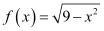Ans: (i) f(x) = –|x|, x ∈ R

We know that |x| =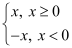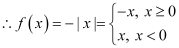Since f(x) is defined for xR, the domain of f is R.

It can be observed that the range of f(x) = –|x| is all real numbers except positive real numbers.

∴The range of f is (–, 0].

(ii)Since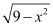is defined for all real numbers that are greater than or equal to –3 and less than or equal to 3, the domain of f(x) is {x : –3 ≤ x ≤ 3} or [–3, 3].

For any value of x such that –3 ≤ x ≤ 3, the value of f(x) will lie between 0 and 3.

∴The range of f(x) is {x: 0 ≤ x ≤ 3} or [0, 3].

Ques 3: A function f is defined by f(x) = 2x – 5. Write down the values of

(i) f(0),                     (ii) f(7),                  (iii) f(–3)

Ans: The given function is f(x) = 2x – 5.

Therefore,

(i) f(0) = 2 × 0 – 5 = 0 – 5 = –5

(ii) f(7) = 2 × 7 – 5 = 14 – 5 = 9

(iii) f(–3) = 2 × (–3) – 5 = – 6 – 5 = –11

Ques 4:The function ‘t’ which maps temperature in degree Celsius into temperature in degree Fahrenheit is defined by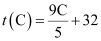.

Find (i) t (0)             (ii) t (28)         (iii) t (–10)         (iv) The value of C, when t(C) = 212

Ans: The given function is.

Therefore,

(i)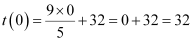(ii)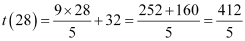(iii)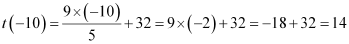(iv) It is given that t(C) = 212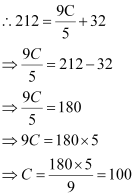Thus, the value of t, when t(C) = 212, is 100.

Ques 5: Find the range of each of the following functions.

(i) f(x) = 2 – 3x, x ∈ R, x > 0.

(ii) f(x) = x2 + 2, x, is a real number.

(iii) f(x) = x, x is a real number

Ans:  (i) Let x > 0

⇒ 3x > 0

⇒ 2 –3x < 2

f(x) < 2

∴Range of f = (–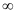, 2)

(ii) Given f (x) = x2 + 2, x is a real number
We know x2≥ 0 ⇒ x2 + 2 ≥ 0 + 2
⇒ x2 + 2 > 2 ∴ f (x) ≥ 2
∴ The range of f (x) is [2, ∞).

(iii) Given f (x) = x, x is a real number.
Let y =f (x) = x ⇒ y = x
∴ Range of f (x) = Domain of f (x)
∴ Range of f (x) is R.

Offer running on EduRev: Apply code STAYHOME200 to get INR 200 off on our premium plan EduRev Infinity!

## Mathematics (Maths) Class 11

158 videos|186 docs|161 tests

,

,

,

,

,

,

,

,

,

,

,

,

,

,

,

,

,

,

,

,

,

;